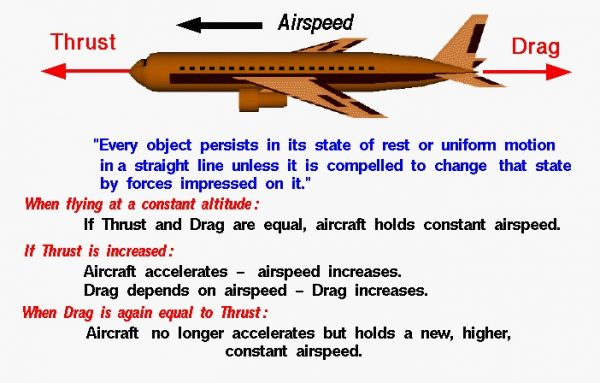# Aircraft Motion – Newton’s First Law## Newton’s Laws of Motion

Sir Isaac Newton first presented his three laws of motion in the “Principia Mathematica Philosophiae Naturalis” in 1686. His first law states that every object remains at rest or in uniform motion in a straight line unless compelled to change its state by the action of an external force. This is normally taken as the definition of inertia. The key point here is that if there is no net force resulting from unbalanced forces acting on an object (if all the external forces cancel each other out), then the object maintains a constant velocity. If that velocity is zero, then the object remains at rest. And if an additional external force is applied, the velocity changes because of the force.

## Motion of an Aircraft

An aircraft in flight is a particularly good example of the first law of motion. There are four major forces acting on an aircraft; lift, weight, thrust, and drag. If we consider the motion of an aircraft at a constant altitude, we can neglect the lift and weight. A cruising aircraft flies at a constant airspeed and the thrust exactly balances the drag of the aircraft. This is the first part sited in Newton’s first law; there is no net force on the airplane and it travels at a constant velocity in a straight line.

## Drag

Now if the pilot changes the thrust of the engine, the thrust and drag are no longer in balance. If the thrust is increased, the aircraft accelerates and the velocity increases. This is the second part sited in Newton’s first law; a net external force changes the velocity of the object. The drag of the aircraft depends on the square of the velocity. So the drag increases with increased velocity. Eventually, the new drag equals the new thrust level and at that point, the forces again balance out, and the acceleration stops. The airplane continues to fly at a new constant velocity that is higher than the initial velocity. We are again back to the first part of the law with the aircraft traveling at a constant velocity.

Remember, in this simple example, that we are only considering the motion of the aircraft in a horizontal direction; we are neglecting any effects of the thrust on weight or on lift. On real aircraft, increasing the throttle setting increases the fuel usage and decreases the weight, and the increase in velocity increases the lift as well as the drag. Each of these changes affect the vertical motion of the aircraft, but to simplify our understanding of Newton’s laws, we are neglecting these effects in this example.

## Thrust

It’s important to point out the role of engine thrust in this example. Thrust is used to accelerate the aircraft, to change its velocity, and thrust is used to balance the drag when the aircraft is cruising at a constant velocity. We normally think of only the first role – acceleration. For fighter planes high thrust is desirable. But some aircraft, like jet airliners, spend most of their existence in cruising flight balancing the drag. In that role engine efficiency (low fuel usage) is desirable. Depending on the mission of the aircraft, different types of engines are designed to provide high thrust or high efficiency.

Provide feedback